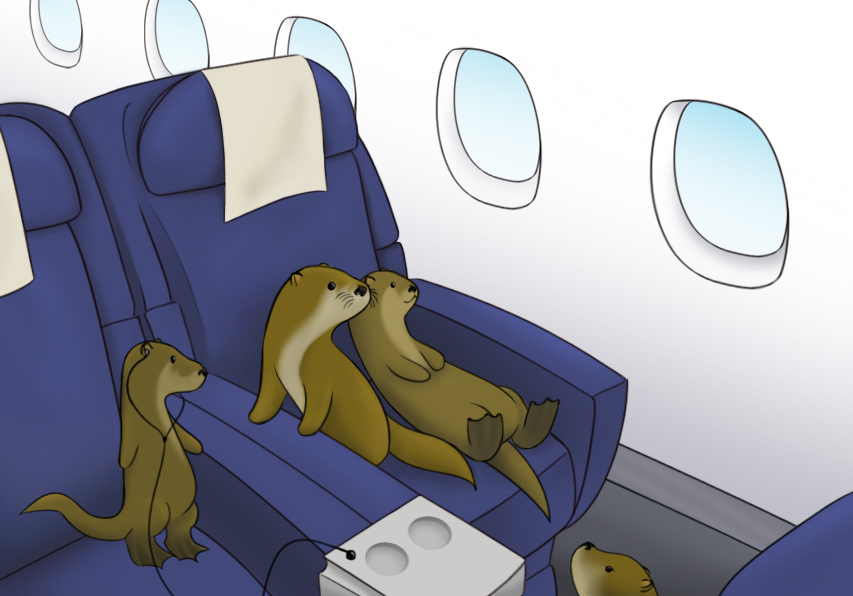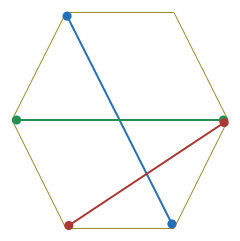Probability

# Discrete Probability: Level 2 ChallengesThere are 100 people in line to board a plane with 100 seats. The first person has lost his boarding pass, so he takes a random seat.

Everyone that follows takes their assigned seat if it's available, but otherwise takes a random unoccupied seat. What is the probability the last passenger ends up in his/her assigned seat, as a decimal?

Alex tells the truth 70% of the time while Blake tells the truth 80% of the time, independently of each other. They are shown an apple and asked "Is it an apple or a banana?". What is the probability that they will give different answers?In a convex hexagon, two diagonals are drawn at random. What is the probability that the diagonals intersect inside the hexagon at 1 point?Two players, Nihar and I, are playing a game in which we alternate tossing a fair coin and the first player to get a head wins. Given that I toss first, the probability that Nihar wins the game is $\dfrac{\alpha}{\beta}$, where $\alpha$ and $\beta$ are coprime positive integers.

Find $\alpha + \beta$.Out of four machines, Titus knows that exactly two are faulty. He wants to test them one by one in a random order (without replacement) until he can identify the 2 faulty machines. Find the probability that exactly two tests are needed?

×